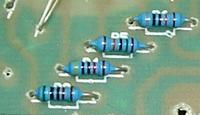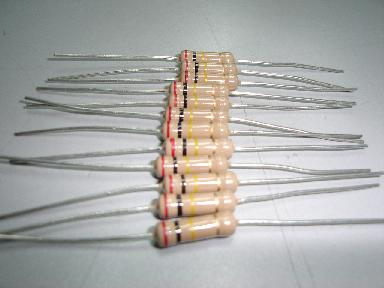## Calculation Of Five Band Resistor Color CodeIn computer monitor repair,  i came across five band resistor color code. So what is the purpose of five band resistors in electronic circuit? The purpose of using the five colour band resistor in a circuit is to provide a more precise value compare to the four color band resistor. Take this as an example, in order to get the value of 33.8 kilo ohm, with four colors band resistor you will not be able to calculate the value.

The most you can get is 33 kilo ohm (orange, orange, orange, gold). If with the five band resistors you will be able to find it (orange, orange, grey, red, brown) the last color which is brown colour represent 1% tolerance. If you want to know more about how to calculate the five band resistor color code then you may visit  the google search engine and type in resistor colour code, resistor colour coding, resistor color code calculator, or resistor color code chart to know more about how to calculate a five band resistors values.

Remove the analogue multimeter cover and you will understand what i mean about the accuracy of using five band resistor in electronic circuit or board. Most of the resistors inside the analog multimeter are using five color band resistor. Why? Because the measurement that you get whenever you measure current, voltage or ohm, the panel will show you the nearest value. For example, if you measure a 3 volt battery the pointer will point to may be 2.9 volt or 3.1 volt. If the analog meter was designed using four band resistor, the result that you gets may be 2.5 volt, 3.5 volt or even 4 volt. In other words the use of five color band resistor is to make a circuit more accurate and output the desire result as what the engineers want it to be.Five band resistors in an analog multimeter

There's a interesting five color band resistor which is very unique. In fact i have already measure quite a number of them. I will reveal the calculation about this unique five color band resistor to you. The colors are orange, orange, red, gold, white. If you take a five band resistor calculation formula to find the value i doubt you could find the value. Actually it is a four color band resistor. Just ignore the white color band and proceed to calculate using the four color band formula and you will definetely find the answer. As regards the "extra" color bands.  They are in fact for military purposes.

Resistors used in anything built for the military must pass stringent testing and accuracy. The color bands denote MTBF, other wise known as meant time between failure, or more precisely how long the resistor will last until it fails. If this unique five band resistor spoilt you can take a four color band resistor as replacement. In some monitor  circuit designed,  usually there is a B+ variable resistor control located at the secondary side of the power supply where we called it as error sensing circuit.

But nowadays the monitor engineers had changed the variable resistor to a five band resistor. Whenever this resistor value change, all the output of the secondary voltage will increased and caused the monitor to goes into shutdown mode. If you came across this type of monitor symptom, the first thing you should do is to measure the five band resistor in the error sensing circuit.Four band resistors color code